# Edexcel A Level Chemistry:复习笔记3.3.6 Addition Polymerisation

• Addition polymerisation is one of the most important addition reactions of alkenes which form the basis of the plastics industry
• Addition polymerisation is the reaction in which many monomers containing at least one C=C double bond form long chains of polymers as the only product
• Just like in other addition reactions of alkenes, the π-bond in each C-C bond breaks and then the monomers link together to form new C-C single bonds

• A polymer is a long-chain molecule that is made up of many repeating units
• The small, reactive molecules that join together to form the polymer are called monomers
• A polymerisation reaction can be represented by a general formula or by using displayed formulae
• Eg. poly(ethene) and poly(chloroethene) (also known as PVC) are polymers made up of the ethene and chloroethene monomers respectively and are commonly used in making plastics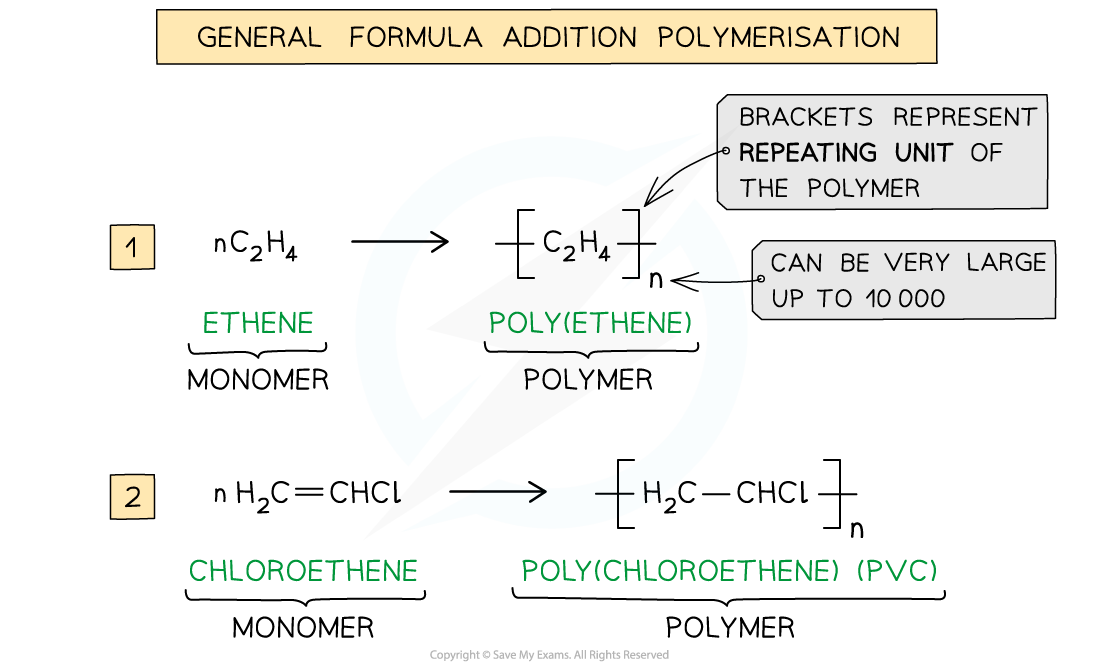The general formulae of the addition polymerisation of ethene (1) and chloroethene (2)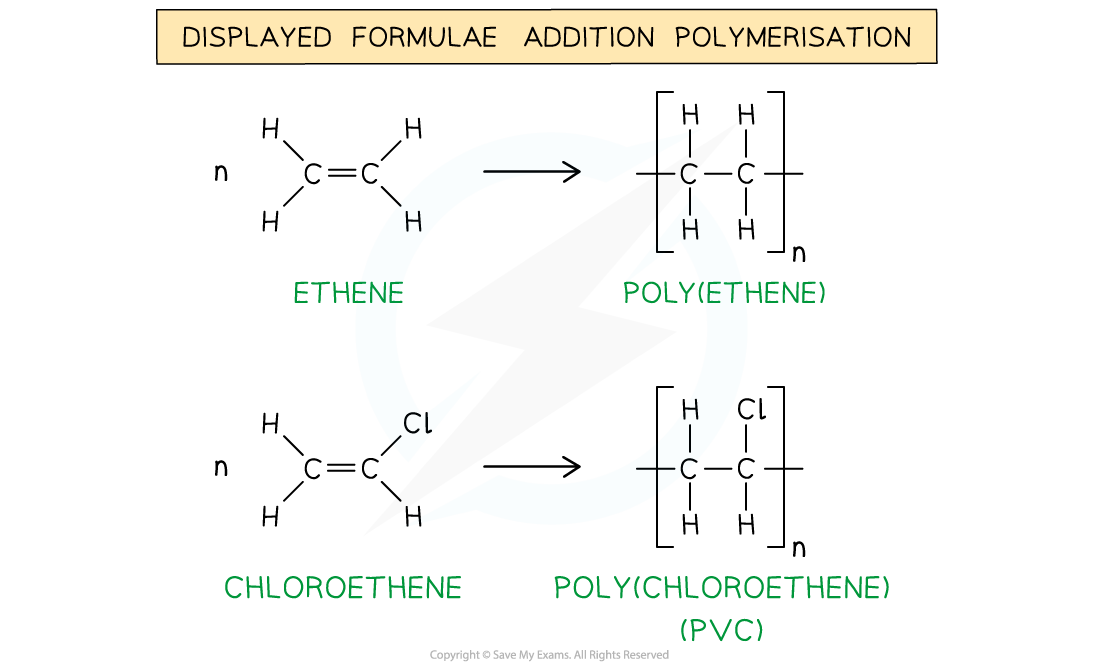The displayed formulae of the addition polymerisation of ethene (1) and chloroethene (2)

• Just like any other addition reaction of alkenes, addition polymerisation gives only one product

#### Deducing repeat units

• A repeat unit is the smallest group of atoms that when connected one after the other make up the polymer chain
• It is represented by square brackets in the displayed and general formula

• In poly(alkenes) (such as poly(ethene)) and substituted poly(alkenes) (such as PVC) made of one type of monomer the repeating unit is the same as the monomer except that the C=C double bond is changed to a C-C single bond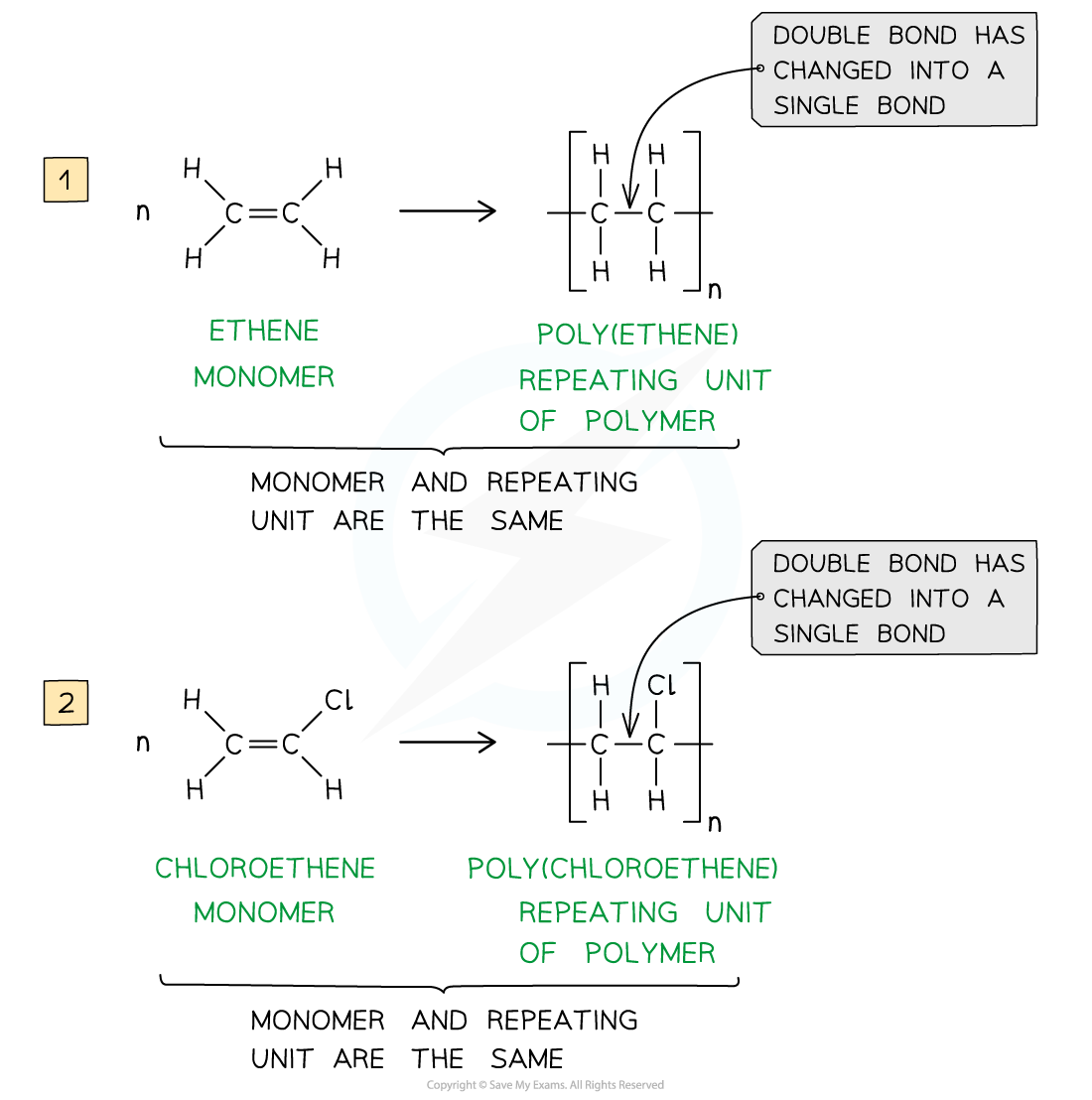The repeating units of poly(ethene) and poly(chloroethene) are similar to their monomer except that the C=C bond has changed into a C-C bond

#### Worked Example

Identifying monomers

Identify the monomers present in the given sections of addition polymer molecules: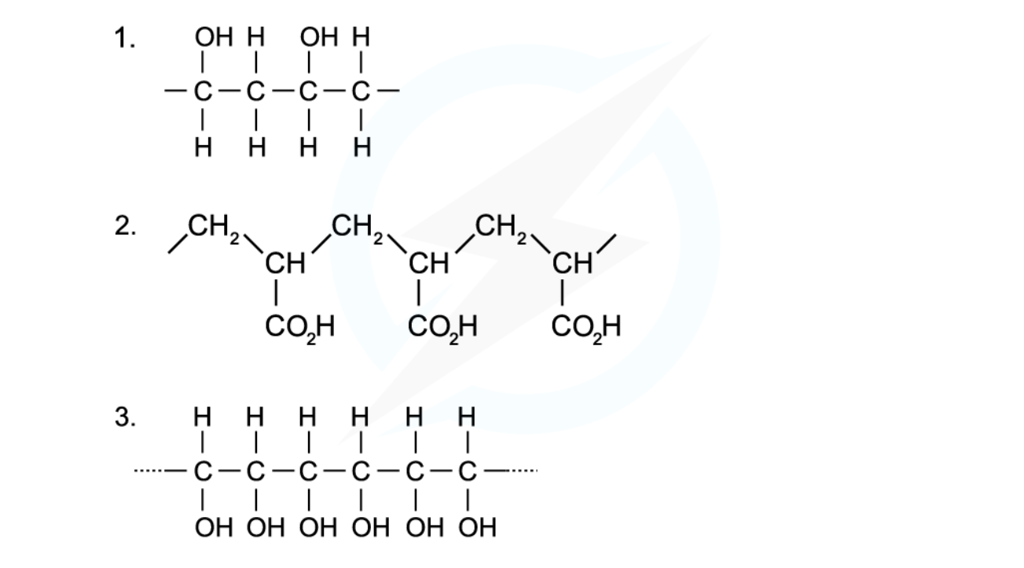• When ethenol, CH(OH)=CH2, is polymerised, the C=C double bond opens to produce a repeating unit of -CH(OH)-CH2- This gives the polymer poly(ethenol)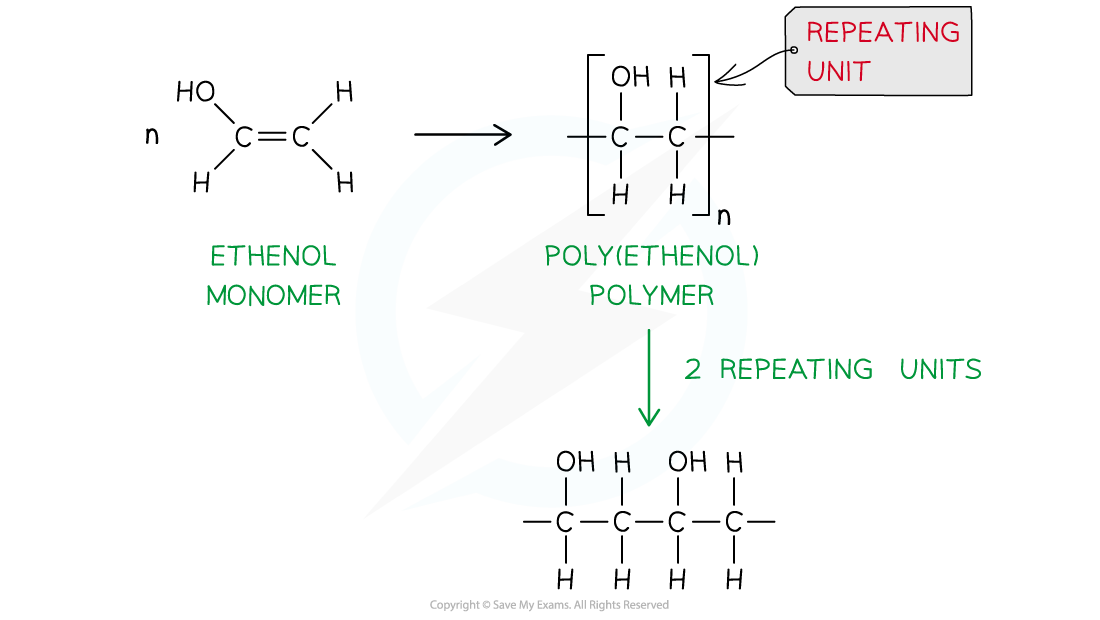• To find the monomer, first the repeating unit should be deduced. Repeating units have only 2 carbons in the polymer main chain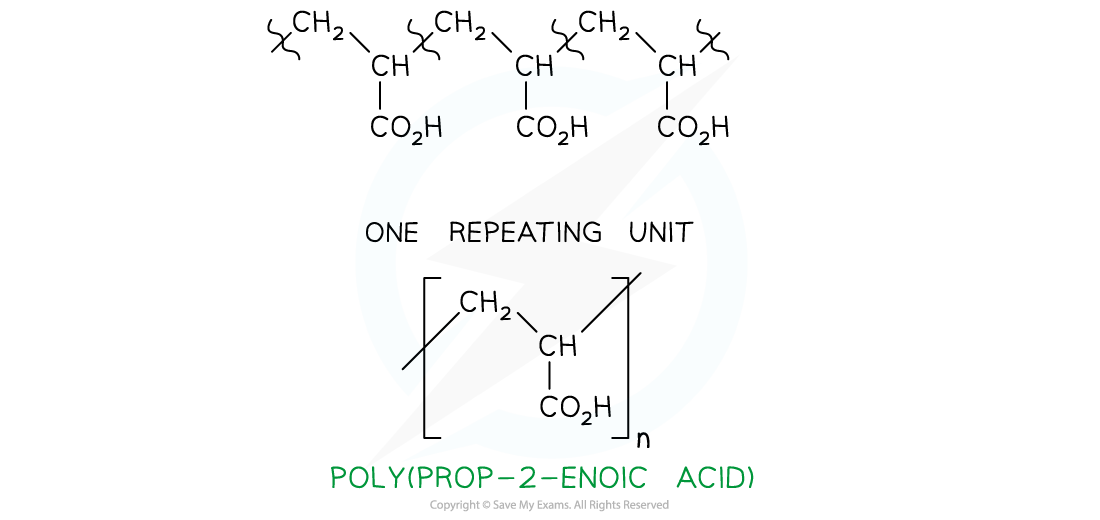• Since the repeating unit is now found, it can be concluded that the monomer is prop-2-enoic acid• Again, the repeating unit only has 2 carbons in the polymer chain which in this case are two carbon atoms that each contain one OH group
• Thus, when ethene-1,2-diol, CH(OH)=CH(OH), is polymerised, the C=C double bond opens to produce a repeating unit of  -CH(OH)-CH(OH)- which gives the polymer poly(ethene-1,2-diol)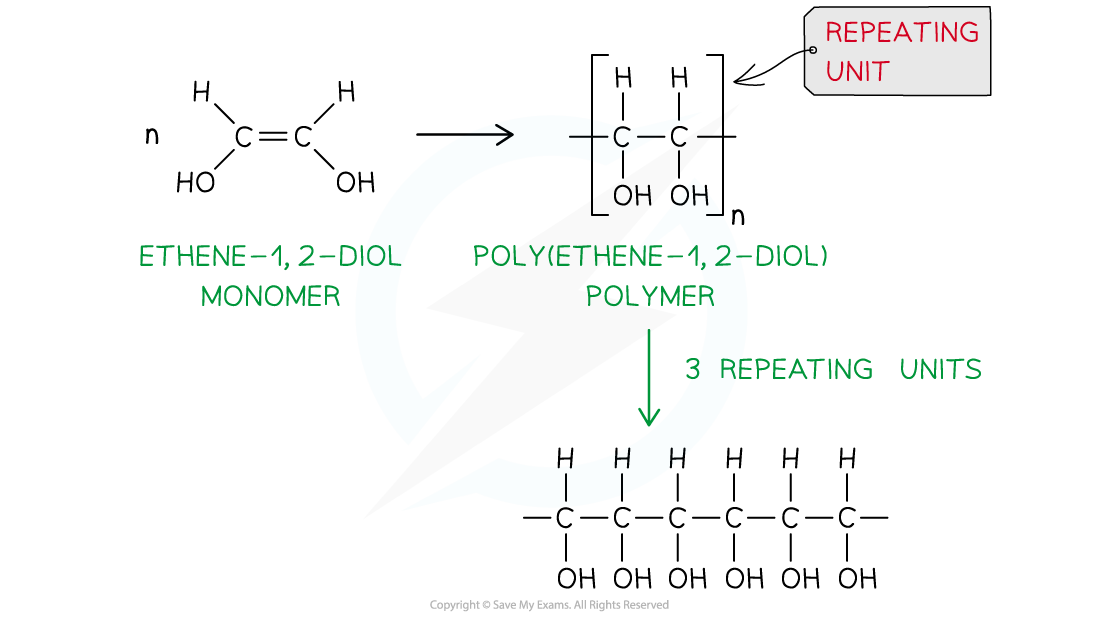#### Exam Tip

The section of the polymer chain shown inside the square brackets by the structural or displayed formula is the repeat unit and not the monomer. The monomer is the same as the repeat unit except for that it has C=C bonds instead of C-C bonds